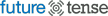# Why You Should Be Excited About the New Record for the Largest Prime Number

Prime numbers—integers that are divisible only by themselves and 1—are the easiest path into understanding both rigor and mysticism in mathematics. Euclid’s proof that there is an infinite number of prime numbers is both one of the simplest mathematical proofs and one of the oldest. Late last month, Curtis Cooper of the University of Central Missouri moved one small step closer to Euclid’s infinity, when he announced that 257,885,161-1 is prime. This is now the largest known prime number, eclipsing the previous record-holder, which had been discovered at UCLA in 2008. The new number has 17,425,170 digits—just writing them down makes for a 22.45-megabyte text file. The UCLA number had knocked an earlier number of Cooper’s, from 2006, out of the record books. With apologies to the Magnetic Fields, the book of primes is long and boring, but an addition to that book is a good chance to look for the music within it.

Cooper and a group at UCLA have been swapping records as part of a common effort called GIMPS, the Great Internet Mersenne Prime Search. Mersenne primes are numbers like 3=22-1 , 7=23-1 or 31=25-1 that are 1 less than a power of 2. The vast majority of such numbers are not prime, but they are good candidates to check for primality nonetheless. All of the 10 largest known primes are Mersenne.

Though mathematicians don’t know whether a given large number is prime until they check, the statistical distribution of primes is well understood. It was one of the central problems of 19th-century mathematics. Many giants of mathematical history—Euler, Legendre, Dirichlet, Gauss, and Riemann among them—worked toward a result describing that distribution, which would become known as the Prime Number Theorem. The remarkable thing about many of these efforts is that they created extremely surprising links between discrete questions—how integers behave—and analytic questions—how continuous functions of real numbers act.

Riemann’s paper On the Number of Prime Numbers Less Than a Given Quantity is often cited by mathematicians as one of the most significant in the history of the field. (For a lovely and readable popular history of Riemann and his mathematical ideas, pick up a copy of Prime Obsession; I interviewed the book’s author here.) But Riemann didn’t prove the Prime Number Theorem, which places rigorous bounds on where large primes can be found. The proof took until 1896, when Jacques Hadamard and Charles Jean de la Vallée-Poussin, French and Belgian mathematicians respectively, independently proved it.

For decades, the behavior of prime numbers was among the central intellectual and aesthetic questions of mathematics, but not one with great practical import. That changed in the last several decades, after Ron Rivest, Adi Shamir, and Leonard Adelman, a trio of young MIT professors, published a paper describing a new cryptographic algorithm in 1977. Their idea was revolutionary (though it was provoked by an earlier paper of Whitfield Diffie and Martin Hellman, at Stanford). The RSA algorithm (after the initials of Rivest, Shamir, and Adelman) was the first system of public-key cryptography, which makes it easy to encrypt messages, and hard to decrypt them, and is the underpinning of modern e-commerce. It depends on knowledge of large prime numbers. As a practical matter, the numbers used are not normally nearly so large as Cooper’s behemoth, which took his computer 39 days to verify as prime, though 3 independent subsequent confirmations took only 3.6, 4.5, and 7.7 days—all still too long to buy something on the Web.

The mathematical fascination with primes comes from how they reveal the hidden structure of the world. Riemann’s paper on primes discussed how their distribution is connected to something that came to be called the Riemann zeta function, which is the subject of Riemann’s hypothesis, probably the most important outstanding problem in mathematics, and the subject of Prime Obsession.